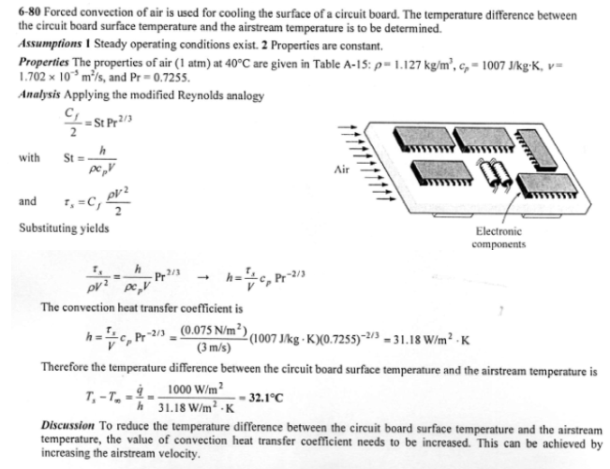heat and mass transfer problems engineering equations heat and mass transfer school homework engineering heat and mass transfer formulas heat and mass transfer solutions to heat and mass transfer problems full solution engineering problem solution heat and mass transfer math problems engineering equations heat and mass transfer school homework engineering solutions to heat and mass transfer formulas heat problems mass problem solutions to transfer problems full solution heat and mass transfer
heat and mass transfer problems engineering equations heat and mass transfer school homework engineering heat and mass transfer formulas heat and mass transfer solutions to heat and mass transfer problems full solution engineering problem solution heat and mass transfer math problems engineering equations heat and mass transfer school homework engineering solutions to heat and mass transfer formulas heat problems mass problem solutions to transfer problems full solution heat and mass transfer
Highalphabet Home Page heat and mass transfer problem solutions Heat and Mass Transfer Page
A 15 cm x 20 cm circuit board is being cooled by forced convection of air at 1 atm. The heat from the circuit board is estimated to be 1000 W/m^2. If the air stream velocity is 3 m/s and the shear stress of the circuit board surface is 0.075 N/m^2, determine the temperature difference between the circuit board surface temperature and the airstream temperature. Evaluate the fluid properties at 40 C.A 15 cm x 20 cm circuit board is being cooled by forced convection of air at 1 atm. The heat from the circuit board is estimated to be 1000 W/m^2. If the air stream velocity is 3 m/s and the shear stress of the circuit board surface is 0.075 N/m^2, determine the temperature difference between the circuit board surface temperature and the airstream temperature. Evaluate the fluid properties at 40 C.